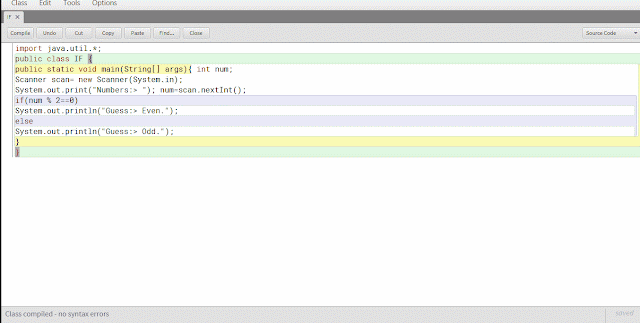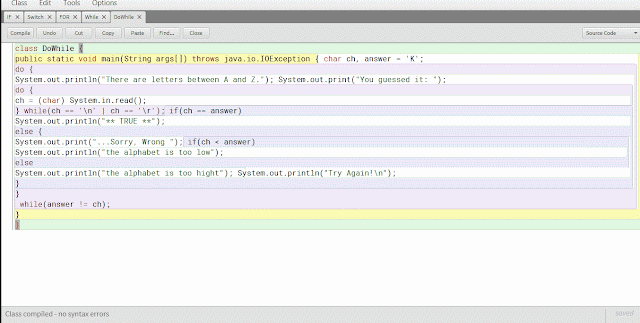Controller Statement JAVA Program

Statement Control Program

In this section we will study the statements that control the flow of the program, namely branching (selection) and process repeatability.

Statement Branching

Branching in Java using two types namely if and switch statements.

if statement

Format syntax branching using the if statement is:
if (expression) {
statement;
}
Else
{
statements other;
}
Examples of java program using IF:
 `01` `import` `java.util.``*``;`
 `02` `public ``class` `IF {`
 `03` `public static void main(String[] args){ ``int` `num;`
 `04` `Scanner scan``=` `new Scanner(System.``in``);`
 `05` `System.out.``print``(``"Numbers:> "``); num``=``scan.nextInt();`
 `06` `if``(num ``%` `2``=``=``0``)`
 `07` `System.out.println(``"Guess:> Even."``);`
 `08` `else`
 `09` `System.out.println(``"Guess:> Odd."``);`
 `10` `}`
 `11` `}`coding result, in the example I insert 4 to test even numbers and odd numbers 5 to test

switch Statement

The switch statement provides a multiway branching, so that the program can select several alternatives. The format switch syntax is as follows:
switch (expression) {case constant1:
statement sequence
break;
constant2 case: statement sequence
break;
constant3 case: statement sequence
break;
...
default:
statement sequence
}
Examples coding java programs using the switch:
 `01` `public ``class` `Switch {`
 `02` `public static void main(String args[]) { ``int` `i;`
 `03` `for``(i``=``0``; i<``10``; i``+``+``)`
 `04` `switch(i) {`
 `05` `case ``0``:`
 `06` `System.out.println (i ``+` `" is zero"``); ``break``;`
 `07` `case ``1``:`
 `08` `System.out.println (i ``+` `" is one"``); ``break``;`
 `09` `case ``2``:`
 `10` `System.out.println (i ``+` `" is two"``); ``break``;`
 `11` `case ``3``:`
 `12` `System.out.println (i ``+` `" is three"``); ``break``;`
 `13` `case ``4``:`
 `14` `System.out.println (i ``+` `" is four"``); ``break``;`
 `15` `default:`
 `16` `System.out.println (i ``+` `" is five or more"``);`
 `17` `}`
 `18` `}`
 `19` `}`coding result, in the above example use case switch to display the words of a number

Repetition Statement

Some repetition is used Java statements include is for, while and do- while.

Statement Loop (for)

Forms to use for repetition statement is:
for (initialization; condition; iteration)
{
statement sequence
}

Initialization, typically used to provide an initial value for the control variable in the loop.
Condition, is a boolean expression that determines whether the loop will be repeated or not.
Iteration, is an expression that defines the amount by which the value loop controllers will change every time repetition.

Examples of uses for coding java programs:
 `01` `class` `FOR {`
 `02` `public static void main(String args[]) { double num, sroot, rerr;`
 `03` `for``(num ``=` `1.0``; num < ``100.0``; num``+``+``) { sroot ``=` `Math.sqrt(num);`
 `04` `System.out.println (``"The square root of "` `+` `num ``+` `" is "` `+` `sroot);`
 `05` `/``/` `compute rounding error`
 `06` `rerr ``=` `num ``-` `(sroot ``*` `sroot);`
 `07` `System.out.println (``"Rounding error is "` `+` `rerr); System.out.println ();`
 `08` `}`
 `09` `}`
 `10` `}`coding result, in the example above is an example of using for to repeat the program seek to know the square root of 1-100

Loop Statement (while)

Another form of repetition in Java process is using while statement. Format syntax is:
while (condition) statement;
statement can be either a single statement or statement within the block. While the condition defines conditions that control the flow of repetition or in the form of a Boolean expression that repetition will continue while the condition is true.

Examples coding Java programs using a while loop:
 `01` `class` `While {`
 `02` `public static void main(String args[]) { ``int` `e;`
 `03` `int` `result;`
 `04` `for``(``int` `i``=``0``; i < ``10``; i``+``+``) { result ``=` `1``;`
 `05` `e ``=` `i;`
 `06` `while``(e > ``0``) {`
 `07` `result ``*``=` `2``; e``-``-``;`
 `08` `}`
 `09` `System.out.println (``"2 rank "` `+` `i ``+` `" is "` `+` `result);`
 `10` `}`
 `11` `}`
 `12` `}`coding results, examples of the program is the use of repetition while to figure out the results of a number 2 rank 0-10

Statement Loop (do-while)

The statement uses the do-while loop has the following syntax format:
do { statements; } While (condition);
The pattern of grooves do-while almost the same while only this format would do repetition first and then check whether the conditions should next or not.

Examples of program coding java do-while:
 `01` `class` `DoWhile {`
 `02` `public static void main(String args[]) throws java.io.IOException { char ch, answer ``=` `'K'``;`
 `03` `do {`
 `04` `System.out.println(``"There are letters between A and Z."``); System.out.``print``(``"You guessed it: "``);`
 `05` `do {`
 `06` `ch ``=` `(char) System.``in``.read();`
 `07` `} ``while``(ch ``=``=` `'\n'` `| ch ``=``=` `'\r'``); ``if``(ch ``=``=` `answer)`
 `08` `System.out.println(``"** TRUE **"``);`
 `09` `else` `{`
 `10` `System.out.``print``(``"...Sorry, Wrong "``); ``if``(ch < answer)`
 `11` `System.out.println(``"the alphabet is too low"``);`
 `12` `else`
 `13` `System.out.println(``"the alphabet is too hight"``); System.out.println(``"Try Again!\n"``);`
 `14` `}`
 `15` `}`
 `16` ` ``while``(answer !``=` `ch);`
 `17` `}`
 `18` `}`coding result, in the above example is a program using do-while to guess the letters to answer the letter is found if any of the program will continue to repeat until we input the correct letter, answers letters on the right example is K

Break can also be used instead of a goto statement that jumps to a label, such as coding examples below:
 `01` `class` `BreakGoto {`
 `02` `public static void main(String args[]) { ``int` `i;`
 `03` `for` `(i ``=` `1``; i <``4``; i ``+``+``) {ONE: {`
 `04` `TWO: {`
 `05` `THREE: {`
 `06` `System.out.println (``"\n is the value i is"` `+` `i); ``if` `(i ``=``=` `1``)`
 `07` `break` `ONE; ``if` `(i ``=``=` `2``)`
 `08` `break` `TWO; ``if` `(i ``=``=` `3``)`
 `09` `break` `THREE; System.out.println (``"will not be printed"``);`
 `10` `}`
 `11` `System.out.println (``"After block three."``);`
 `12` `}`
 `13` `System.out.println (``"After block two."``);`
 `14` `}`
 `15` `System.out.println (``"After block one."``);`
 `16` `}`
 `17` `System.out.println (``"After."``);`
 `18` `}`
 `19` `}`

continue statement

This statement is the opposite of a break, continue statement is used to continue the direct repetition, such as coding examples below:
 `1` `public ``class` `Continue {`
 `2` `public static void main(String args[]) { ``int` `i;`
 `3` `for``(i ``=` `0``; i<``=``10``; i``+``+``) {`
 `4` `if``((i``%``2``) !``=` `0``)`
 `5` `continue``; System.out.println(i);`
 `6` `}`
 `7` `}`
 `8` `}`coding results, will display the number of the 0-10 to continue for even numbers% 2